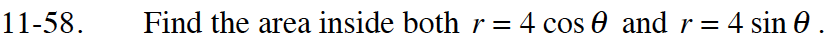### Home > CALC > Chapter 11 > Lesson 11.2.2 > Problem11-58

11-58.

Find the area inside both r = 4 cos θ and r = 4 sin θ . Homework Help ✎Determine the bounds by solving 4cos(θ) = 4sin(θ).

By looking at the graph, you can calculate the area inside of r = sin(θ) for 0 ≤ θπ/4 and double it.